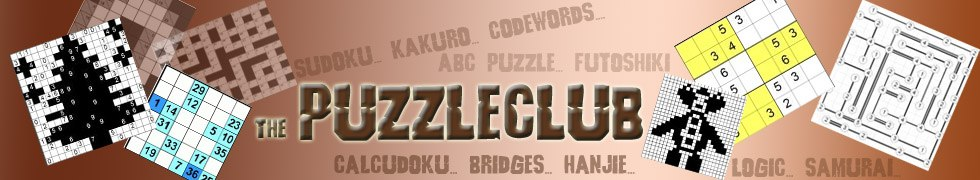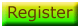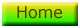Number Square Puzzles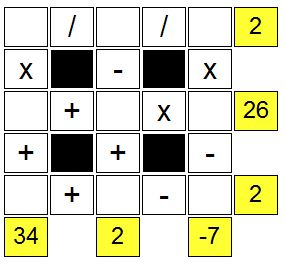The number square is an enjoyable puzzle with simple rules. You can see a sample of a number square solution to the right, which we'll use to explain the rules below.

The first thing you'll notice, is that there is a 3 x 3 grid of empty cells: each of these must contain the numbers from 1 - 9 exactly once.

You'll also see there are certain symbols between the numbers... as you might expect, these are used to form sums whose totals must match what you see at the end of each row or column, which is a total.

The symbols are + for addition, - for subtraction, x for multiplication and the / sign which is the only one you may be unfamiliar with means that you should divide.

Please note that the sums run from left to right or top to bottom, so you should ignore strict mathematical order which would for instance always have you do the multiplication part of a sum before the subtraction part assuming there were no brackets: for simplicity these puzzles all follow the same order of performing calculations left to right and top to bottom.

You can play a Sample Number Square online. There are some tips on how to solve the puzzles on that page.

If you like number squares, or maths squares as they are sometimes known, then you'll be pleased to here that we have added a fantastic 100 maths square puzzles to the members section of the site. If you're a member, then you'll see these puzzles below right now:

Members Puzzles

Just pick any of the 100 number square puzzles below to play... have fun!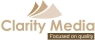©   |     |